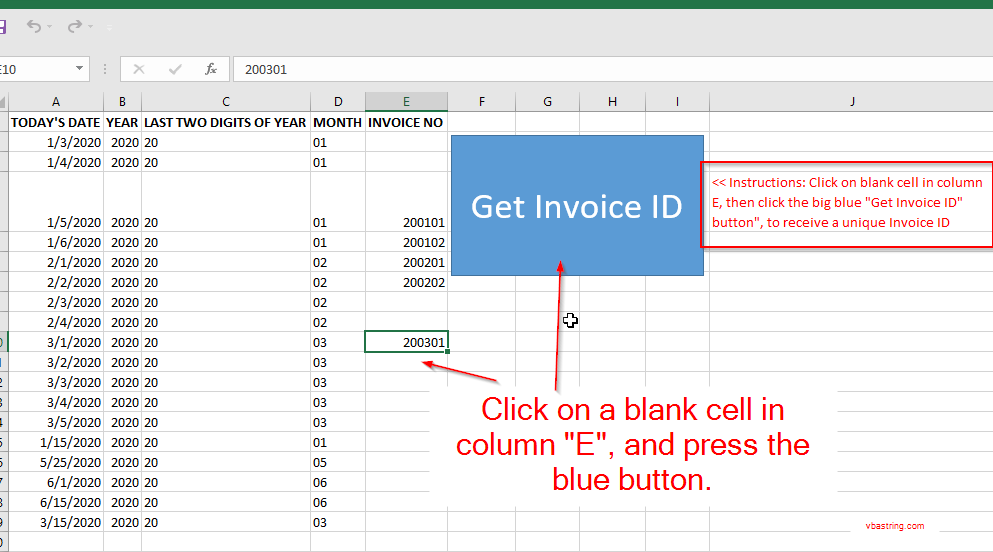# Automatic Invoice Number Generator Excel VBA Project

This post is actually in response to an Excel VBA question.

Basically it can be summarized by titling it “automatic invoice number generator excel”

That is what this is:This can be filled out with user form, but all the data ultimately gets entered into the underlying spreadsheet so we’ll skip the user form part (let me know if you actually want to see it).

Column A is just a date (“TODAY’S DATE”).
Column B is a formula based on column A, “=YEAR(A3)”. (“YEAR”)
Column C is a formula based on column B, “=RIGHT(B2,2)”. (“LAST TWO DIGITS OF YEAR”)
Column D is just the “Month” function based on Column A, but the single digit months are padded “=IF(LEN(MONTH(A2))<10,"0" & MONTH(A2),MONTH(A2))". (“MONTH”)

and finally Column “E” is the “INVOICE NO” generated with the following code:

The real “work horse” here is the function “GetNextInvoiceID”.

It uses a for loop to search Column “E” for evidence that the invoice number was used, and it will use the next higher number instead.

What’s also worth noting is that you can use your normal Excel worksheet functions in your VBA by using, the Application.Worksheetfunction syntax: ex. Application.WorksheetFunction.CountIf

Let me know if you have questions.